Worksheets and No Prep Teaching Resources
Math Worksheets

# Hundreds Chart Pieces Puzzle Worksheets Puzzle Workbooks for Kids - Updated Each Month!

Learning how to count to one hundred is a basic skill that is fun to teach with puzzles. These fascinating worksheets will help students master this essential skill while having a fantastic time! Versatile worksheets that can be customized to meet students' individual needs and skill levels are winners for any classroom!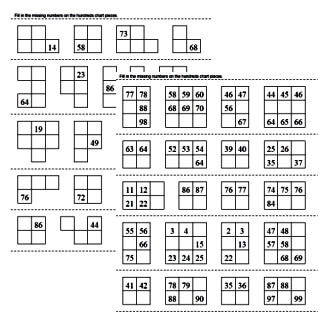Complete Hundreds Chart (1 to 100) Math Learning Center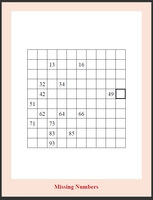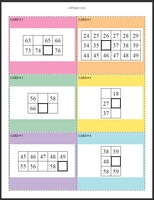(complete chart on each card) Easiest (Grades 1-3) (complete chart on each card) A few more blanks: Write one of the missing numbers (Grades 1-3) (complete chart on each card) Most Difficult: Numbers not next to blank (Grades 1-3)

Numbers Chart Math Learning Center - 5 rows on each card(5 rows on each card) Easiest (Grades 1-3) (5 rows on each card) A few numbers not shown (Grades 1-3) (5 rows on each card) More Difficult: will have blank boxes next to the number that needs to be written (Grades 1-3) (5 rows on each card) Only one number is on each chart - number can be in the same row as the number to write (Grades 1-3) (5 rows on each card) Most Difficult: Only one number is on each chart and number cannot be in the same row as the number to write (Grades 1-3)

Numbers Chart Math Learning Center - 3 to 4 rows on each card(3 to 4 rows on each card) Easiest (Grades 1-3) (3 to 4 rows on each card) A few numbers not shown (Grades 1-3) (3 to 4 rows on each card) More Difficult: will have blank boxes next to the number that needs to be written (Grades 1-3) (3 to 4 rows on each card) Only one number is on each chart - number can be in the same row as the number to write (Grades 1-3) (3 to 4 rows on each card) Most Difficult: Only one number is on each chart and number cannot be in the same row as the number to write (Grades 1-3)

Numbers Chart Math Learning Center - 2 to 3 rows on each card(2 to 3 rows on each card) Easiest (Grades 1-3) (2 to 3 rows on each card) A few numbers not shown (Grades 1-3) (2 to 3 rows on each card) More Difficult: will have blank boxes next to the number that needs to be written (Grades 1-3) (2 to 3 rows on each card) Only one number is on each chart - number can be in the same row as the number to write (Grades 1-3) (2 to 3 rows on each card) Most Difficult: Only one number is on each chart and number cannot be in the same row as the number to write (Grades 1-3)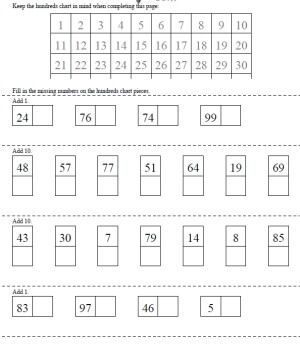Adding 1 or Adding 10 (partial hundreds chart on top of page)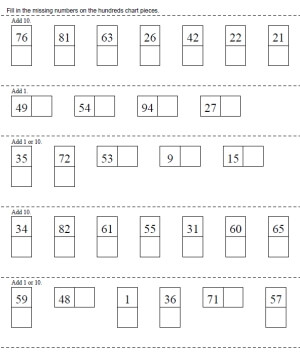Adding 1 or Adding 10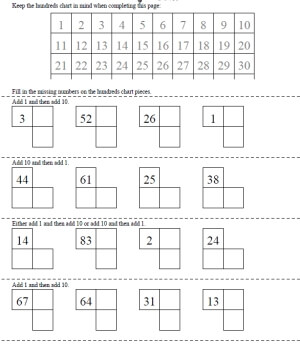Adding 1 and then Adding 10 or Adding 10 and then Adding 1 (partial hundreds chart on top of page) Adding 1 and then Adding 10 or Adding 10 and then Adding 1

Hundreds Chart Pieces Puzzle:  Subtracting 1 or Subtracting 10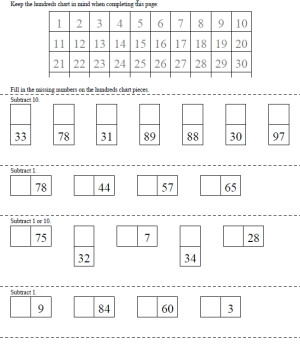Subtracting 1 or Subtracting 10 (partial hundreds chart on top of page) Subtracting 1 or Subtracting 10

Hundreds Chart Pieces Puzzle:  Mix of Addition and Subtraction of 1 or 10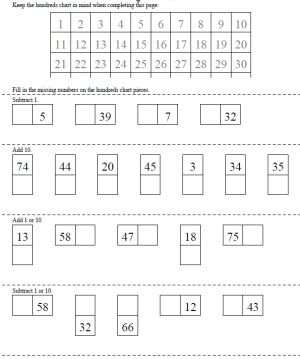Mix of Addition and Subtraction of 1 or 10 (partial hundreds chart on top of page) Mix of Addition and Subtraction of 1 or 10

Hundreds Chart Pieces Puzzle Challenge:  1 Number Given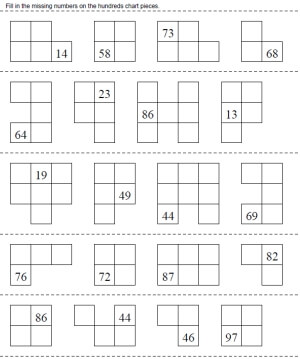Mix of Addition and Subtraction of 1 or 10 (partial hundreds chart on top of page)

Fill in Missing Pieces in a Hundreds Chart

Easiest - Add or Subtract 1:  Missing pieces always have a number to the left or right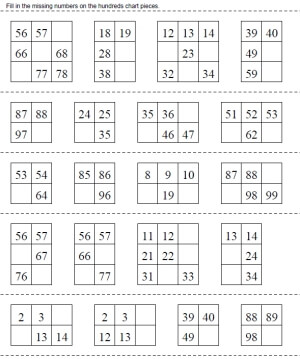One or two blanks 25% of chart will be blank Up to half of the chart will be blank

Add or Subtract 1 or 10:  Missing pieces always have at least one number either to the top, right, left, or bottom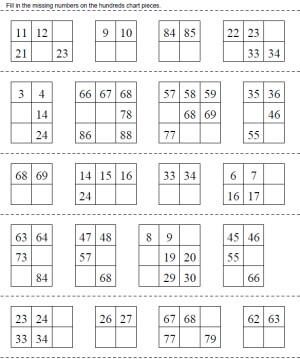One or two blanks 25% of chart will be blank Up to half of the chart will be blank Up to 75% of the chart will be blank

Hundreds Chart Pieces Puzzle - Fill In Blanks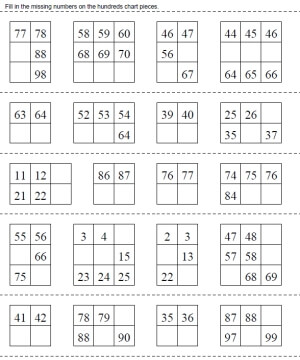One or two blanks 25% to 50% of chart will be blank All blank except for the first two numbers

Hundreds Chart

Counting Chart Puzzle

Have a suggestion or would like to leave feedback?